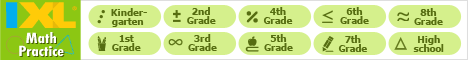Java Games: Flashcards, matching, concentration, and word search.# slope/y-intercept

match the slope (m) or the y-intercept (b) with the equations

AB
y = 3x + 5, find b.5
y = -2x + 5, find m.-2
y = 1/2x - 1, find b.-1
y = 1/2x + 1, find m.1/2
y = x - 5, find m.1
y = x - 5, find b.-5
2y = 4x, find m.2
y = 3x, find b.0This activity was created by a Quia Web subscriber. Learn more about QuiaCreate your own activities